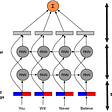# What is the difference between cost function and activation function?

• A cost function is a measure of error between what value your model predicts and what the value actually is. For example, say we wish to predict the value yi for data point xi.
• represent the prediction or output of some arbitrary model for the point xi with parameters θ. One of the many cost functions could be

this function is known as the L2 loss. Training the hypothetical model we stated above would be the process of finding the θ that minimizes this sum.

-An activation function transforms the shape/representation of the data going into it. A simple example could be max(0, xi), a function which outputs 0 if the input xi is negative or xi if the input xi is positive. This function is known as the “ReLU” or “Rectified Linear Unit” activation function. The choice of which function(s) are best for a specific problem using a particular neural architecture is still under a lot of discussions. However, these representations are essential for making high-dimensional data linearly separable, which is one of the many uses of a neural network.

# What is the difference between cost function and loss function?

As mentioned by others, cost and loss functions are synonymous (some people also call it error function). The more general scenario is to define an objective function first, which you want to optimize. This objective function could be to

- maximize the posterior probabilities (e.g., naive Bayes)
- maximize a fitness function (genetic programming)
- maximize the total reward/value function (reinforcement learning)
- maximize information gain/minimize child node impurities (CART decision tree classification)
- minimize a mean squared error cost (or loss) function (CART, decision tree regression, linear regression, adaptive linear neurons, …
- maximize log-likelihood or minimize cross-entropy loss (or cost) function
- minimize hinge loss (support vector machine)

The loss function (or error) is for a single training example, while the cost function is over the entire training set (or mini-batch for mini-batch gradient descent).

Generally cost and loss functions are synonymous but cost function can contain regularization terms in addition to loss function. although it is not always necessary.

Which LOSS AND ACTIVATION function to use?

# LOSS FUNCTION:

Loss function is a method of evaluating “how well your algorithm models your dataset”. If your predictions are totally off, your loss function will output a higher number. If they’re pretty good, it’ll output a lower number. As you tune your algorithm to try and improve your model, your loss function will tell you if you’re improving or not. ‘Loss’ helps us to understand how much the predicted value differ from actual value

Types of Loss function:

1. Regression Loss Function:

Regression models deals with predicting a continuous value for example given floor area, number of rooms, size of rooms, predict the price of the room. The loss function used in the regression problem is called Regression Loss Function”.

2. Binary Classification Loss Functions:

• Binary classification is a prediction algorithm where the output can be either one of two items, indicated by 0 or 1. The output of binary classification algorithms is a prediction score (mostly). So the classification happens based on the threshold the value (default value is 0.5). If the prediction score > threshold then 1 else 0.

3. Multi-class Classification Loss Functions:

• Multi-Class classification are those predictive modeling problems where there are more target variables/class. It is just the extension of binary classification problem.

# COST FUNCTION:

The loss error is computed for a single training example. If we have ‘m’ number of examples then the average of the loss function of the entire training set is called ‘Cost function’.

Cost function (J) = 1/m (Sum of Loss error for ‘m’ examples)

The shape of cost function graph against parameters (W and b) is a cup up parabola with a single minimum value called ‘local optima’.

It is a function that measures the performance of a Machine Learning model for given data. Cost Function quantifies the error between predicted values and expected values and presents it in the form of a single real number. Depending on the problem Cost Function can be formed in many different ways. The purpose of Cost Function is to be either:

• Minimized — then returned value is usually called cost, loss or error. The goal is to find the values of model parameters for which Cost Function return as small number as possible.
• Maximized — then the value it yields is named a reward. The goal is to find values of model parameters for which returned number is as large as possible.
• Given a model using the following formula:

where:

• ŷ — predicted value,
• x — vector of data used for prediction or training,
• w — weight.

# TYPES OF LOSS FUNCTION:

1. Regression Loss Functions…
2. Mean Squared Error Loss
3. Mean Squared Logarithmic Error Loss
4. Mean Absolute Error Loss

2. Binary Classification Loss Functions

1. Binary Cross-Entropy
2. Hinge Loss
3. Squared Hinge Loss

3. Multi-Class Classification Loss Functions

1. Multi-Class Cross-Entropy Loss
2. Sparse Multiclass Cross-Entropy Loss
3. Kullback Leibler Divergence Loss

# Cost functions for Regression problems:

• Mean Error (ME)
• Mean Squared Error (MSE)
• Mean Absolute Error (MAE)
• Root Mean Squared Error (RMSE)
• Categorical Cross Entropy Cost Function.
• Binary Cross Entropy Cost Function.

“THERE ARE MANY BUT I HAVE COVERED A FEW (MOST COMMON)”.

# Mean Absolute Error

Regression metric which measures the average magnitude of errors in a group of predictions, without considering their directions. In other words, it’s a mean of absolute differences among predictions and expected results where all individual deviations have even importance.

where:

• i — index of sample,
• ŷ — predicted value,
• y — expected value,
• m — number of samples in dataset.

Sometimes it is possible to see the form of formula with swapped predicted value and expected value, but it works the same.

# Mean Squared Error

One of the most commonly used and firstly explained regression metrics. Average squared difference between the predictions and expected results. In other words, an alteration of MAE where instead of taking the absolute value of differences, they are squared.

In MAE, the partial error values were equal to the distances between points in the coordinate system. Regarding MSE, each partial error is equivalent to the area of the square created out of the geometrical distance between the measured points. All region areas are summed up and averaged.

The MSE formula can be written like this:

• i — index of sample,
• ŷ — predicted value,
• y — expected value,
• m — number of samples in dataset.

There are different forms of MSE formula, where there is no division by two in the denominator. Its presence makes MSE derivation calculus cleaner.

Calculating derivative of equations using absolute value is problematic. MSE uses exponentiation instead and consequently has good mathematical properties which make the computation of it’s derivative easier in comparison to MAE. It is relevant when using a model that relies on the Gradient Descent algorithm.

# Root Mean Square error (RMSE)

Root Mean Square error is the extension of MSE — measured as the average of square root of sum of squared differences between predictions and actual observations.

# Binary Cross Entropy Loss Function

This cost function originally stems from information theory with the transfer of bits and how much bits have been lost in the process.

Binary cross entropy measures how far away from the true value (which is either 0 or 1) the prediction is for each of the classes and then averages these class-wise errors to obtain the final loss.

We can define cross entropy as the difference between two probability distributions p and q, where p is our true output and q is our estimate of this true output.

This difference is now applied to our neural networks, where it is extremely effective because of their strong usage of probability.

We can see above that p is compared to log-q(x) which will find the distance between the two.

Cross entropy will work best when the data is normalized (forced between 0 and 1) as this will represent it as a probability. This normalization property is common in most cost functions.

# Categorical cross-entropy

It is a loss function that is used for single label categorization. This is when only one category is applicable for each data point. In other words, an example can belong to one class only.

Use categorical crossentropy in classification problems where only one result can be correct.

​​Example:​ In the ​MNIST​​ problem where you have images of the numbers 0,1, 2, 3, 4, 5, 6, 7, 8, and 9. Categorical crossentropy gives the probability that an image of a number is, for example, a 4 or a 9.

where ŷ is the predicted value.

Categorical cross-entropy will compare the distribution of the predictions (the activations in the output layer, one for each class) with the true distribution, where the probability of the true class is set to 1 and 0 for the other classes. To put it in a different way, the true class is represented as a one-hot encoded vector, and the closer the model’s outputs are to that vector, the lower the loss.

# Hinge Loss

The function max(0,1-t) is called the hinge loss function. It is equal to 0 when t≥1. Its derivative is -1 if t<1 and 0 if t>1. It is not differentiable at t=1 . but we can still use gradient descent using any subderivative at t=1.

• Hinge loss is most popular loss function during pre-deep learning era.
• Hinge loss is often used for binary classification problems.
• This type of loss is primarily used in SVM classifiers where the target values are in the set {-1, 1}.
• When using hinge loss it is important to change the class label to -1 and +1.

Hinge Loss not only penalizes the wrong predictions but also the right predictions that are not confident. Hinge loss output the value ranges from -inf to 1. Hinge loss is used for maximum-margin classification. Though hinge loss is not differentiable, it’s convex function which makes it easy to work with usual convex optimizers used in machine learning domain.

# Multi-class Cross Entropy Loss:

• Most time,Multi-class Cross Entropy Loss is used as a loss function. The generalised form of cross entropy loss is the multi-class cross entropy loss.
• M — No of classes
• y — binary indicator (0 or 1) if class label c is the correct classification for input o
• p — predicted probability score of input o belonging to class c.

# Kullback-Liebler Divergence LOSS (KL-Divergence):

KL Divergence is a measure of how a probability one distribution differs from another distribution.

Dkl(P||Q) is interpreted as the information gain when distribution Q is used instead of distribution P .

Dkl(Q||P) is interpreted as the information gain when distribution P is used instead of distribution Q.

Information gain (IG) measures how much “information” a feature gives us about the class.

KL Divergence is also know as “Relative entropy”. Dkl(P||Q) is read as Divergence from Q to P.

1. Dkl(P||Q) !=Dkl(Q||P). Divergence from Q to P is not symmetric to Divergence from P to Q.

Eg: Dkl(P||Q) =0.086 ;Dkl(Q||P)=0.096

It clear from the example that Dkl(P||Q) not symmetric to Dkl(Q||P).

2. Dkl(P||P) =0 meaning both have identical distribution and not information is gained from other distribution.

3.The value of Dkl(P||Q) will be greater than or equal to zero.

So, the goal of the KL divergence loss is to approximate the true probability distribution P of our target variables with respect to the input features, given some approximate distribution Q. This Can be achieved by minimizing the Dkl(P||Q) then it is called forward KL. If we are minimizing Dkl(Q||P) then it is called backward KL.

Forward KL → applied in Supervised Learning

Backward KL → applied in Reinforcement learning

Q.Why KL divergence is not considered as distance metric?

Reason is simple KL divergence is not symmetric.

KL- Divergence is functionally similar to multi-class Cross entropy .

# Huber loss :

The Huber loss function describes the penalty incurred by an estimation procedure f. Huber (1964) defines the loss function piecewise by [^]

This function is quadratic for small values of a, and linear for large values, with equal values and slopes of the different sections at the two points where

. The variable a often refers to the residuals, that is to the difference between the observed and predicted values

so the former can be expanded to<-

• Huber loss is less sensitive to outliers in data than the squared error loss. This function is quadratic for small values of error /residual, and linear for large values. What is that small value for the error to make the function quadratic depends on the “hyperparameter”, 𝛿 (delta), which can be tuned.
• Huber loss approaches MAE when 𝛿 ~ 0 and MSE when 𝛿 ~ ∞ (large numbers). Choice of delta is important because it decide what value you are willing set as an outlier. Error >delta (outlier cases)are minimized with L1 (Reason : Robust to outlier), while error < delta (inlier cases) are minimized “appropriately” with L2.

# ACTIVATION FUNCTION:

An activation function is a very important feature of an artificial neural network , they basically decide whether the neuron should be activated or not.

In artificial neural networks, the activation function defines the output of that node given an input or set of inputs.

# Activation function Types :

• Linear function
• Binary Step function
• Non-Linear function

# Properties that Activation function should hold?

Derivative or Differential: Change in y-axis w.r.t. change in x-axis.It is also known as slope.(Back prop)

Monotonic function: A function which is either entirely non-increasing or non-decreasing.

# Advantage of Non-linear function over the Linear function :

• Differential are possible in all the non -linear function.
• Stacking of network is possible , which helps us in creating the deep neural nets.

# Linear Types:

A linear activation function takes the form:

y=mx+c ( m is line equation represents W and c is represented as b in neural nets so equation can be modified as y=Wx+b)

It takes the inputs (Xi’s), multiplied by the weights(Wi’s) for each neuron, and creates an output proportional to the input. In simple term, weighted sum input is proportional to output.

# Binary Step Function:

Binary step function are popular known as “ Threshold function”. It is very simple function.

The gradient(differential ) of the binary step function is zero,which is the very big problem in back prop for weight updation.

Another problem with a step function is that it can handle binary class problem alone.(Though with some tweak we can use it for multi-class problem)

# Non-linear Types:

The deep learning rocketing to the sky because of the non-linear functions.Most modern neural network use the non-linear function as their activation function to fire the neuron. Reason being they allow the model to create complex mappings between the network’s inputs and outputs, which are essential for learning and modeling complex data, such as images, video, audio, and data sets which are non-linear or have high dimensionality. Differential are possible in all the non -linear function.

Stacking of network is possible , which helps us in creating the deep neural nets.

The Nonlinear Activation Functions are mainly divided on the basis of their range or curves.

# 1. Sigmoid / Logistic

• Smooth gradient, preventing “jumps” in output values.
• Output values bound between 0 and 1, normalizing the output of each neuron.
• Clear predictions — For X above 2 or below -2, tends to bring the Y value (the prediction) to the edge of the curve, very close to 1 or 0. This enables clear predictions.

• Vanishing gradient — for very high or very low values of X, there is almost no change to the prediction, causing a vanishing gradient problem. This can result in the network refusing to learn further, or being too slow to reach an accurate prediction.
• Outputs not zero centered.
• Computationally expensive

# 2. TanH / Hyperbolic Tangent

• Zero centered — making it easier to model inputs that have strongly negative, neutral, and strongly positive values.
• Otherwise like the Sigmoid function.

• Like the Sigmoid function

# 3. ReLU (Rectified Linear Unit)

• Computationally efficient — allows the network to converge very quickly
• Non-linear — although it looks like a linear function, ReLU has a derivative function and allows for backpropagation

• The Dying ReLU problem — when inputs approach zero, or are negative, the gradient of the function becomes zero, the network cannot perform backpropagation and cannot learn

# 4. Leaky ReLU

• Prevents dying ReLU problem — this variation of ReLU has a small positive slope in the negative area, so it does enable backpropagation, even for negative input values
• Otherwise like ReLU

• Results not consistent — leaky ReLU does not provide consistent predictions for negative input values.

# 5. Parametric ReLU

• Allows the negative slope to be learned — unlike leaky ReLU, this function provides the slope of the negative part of the function as an argument. It is, therefore, possible to perform backpropagation and learn the most appropriate value of α.
• Otherwise like ReLU

• May perform differently for different problems.

# 6. Softmax

• Able to handle multiple classes only one class in other activation functions — normalizes the outputs for each class between 0 and 1, and divides by their sum, giving the probability of the input value being in a specific class.
• Useful for output neurons — typically Softmax is used only for the output layer, for neural networks that need to classify inputs into multiple categories.

# 7. Swish (A Self-Gated)Activation Function

Swish is a new, self-gated activation function discovered by researchers at Google. According to their paper, it performs better than ReLU with a similar level of computational efficiency. In experiments on ImageNet with identical models running ReLU and Swish, the new function achieved top -1 classification accuracy 0.6–0.9% higher.

1. Unboundedness: Unlike sigmoid and tanh functions, Swish is unbounded above which makes it useful near the gradients with values near to 0. This feature avoids Saturation as training becomes slow near 0 gradient values.
2. Smoothness of the curve: Smoothness plays an important role in generalization and optimization. Unlike ReLU, Swish is a smooth function which makes it less sensitive to initializing weights and learning rate.
3. Bounded Below: Like most of the activation functions out there, Swish is also bounded below which helps in strong regularization effects. Like ReLU and softplus, Swish produces produces negative outputs for small negative inputs due to its non-monotonicity. The non-monotonicity of Swish increases expressivity and improves gradient flow, which is important considering that many preactivations fall into this range.

# 8. ELU (Exponential Linear Unit)

• ELU is also proposed to solve the problem of dying neuron.
• Zero-centric

• Computationally intensive.
• Similar to Leaky ReLU, although theoretically better than ReLU, there is currently no good evidence in practice that ELU is always better than ReLU.
• f(x) is monotonic only if alpha is greater than or equal to 0.
• f’(x) derivative of ELU is monotonic only if alpha lies between 0 and 1.
• Slow convergence due to exponential function.

# 9. Softmax

The Softmax regression is a form of logistic regression that normalizes an input value into a vector of values that follows a probability distribution whose total sums up to 1. The output values are between the range [0,1] which is nice because we are able to avoid binary classification and accommodate as many classes or dimensions in our neural network model. This is why softmax is sometimes referred to as a multinomial logistic regression.

# 10. Softplus

Softplus function: f(x) = ln(1+e^x). The softplus function is similar to the ReLU function, but it is relatively smoother.

Derivative of the Softplus function is f’(x) is logistic function (1/(1+exp x)).

Both the ReLU and Softplus are largely similar, except near 0 where the softplus is enticingly smooth and differentiable. It’s much easier and efficient to compute ReLU and its derivative than for the softplus function which has log(.) and exp(.) in its formulation.Interestingly, the derivative of the softplus function is the logistic function

# 11. Maxout function

The Maxout activation is a generalization of the ReLU and the leaky ReLU functions. It is a learnable activation function.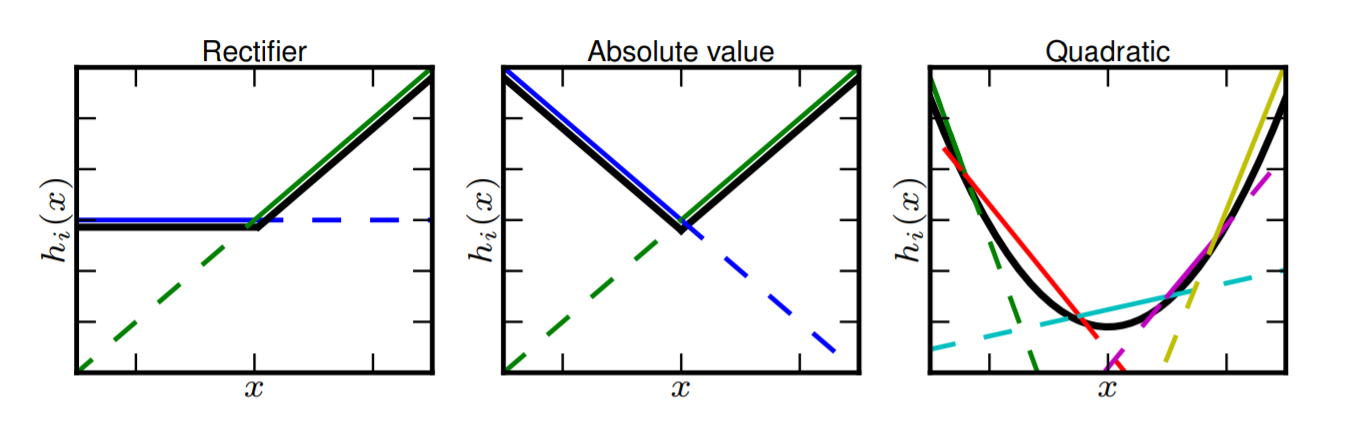Depiction of how the Maxout activation function can implement the ReLU, Absolute function, and approximate the quadratic function. A Maxout unit can approximate arbitrary convex functions

A maxout unit can learn a piecewise linear, convex function with up to k pieces.

So when k is 2, you can implement the ReLU, absolute ReLU, leaky ReLU, etc., or it can learn to implement a new function. If k is let’s say 10, you can even approximately learn the convex function.

When k is 2: the Maxout neuron computes the function max(wT1x+b1,wT2x+b2)max(w1Tx+b1,w2Tx+b2). Both ReLU and Leaky ReLU are a special case of this form (for example, for ReLU we have w1,b1=0w1,b1=0). The Maxout neuron therefore enjoys all the benefits of a ReLU unit (linear regime of operation, no saturation) and does not have its drawbacks (dying ReLU).

However, unlike the ReLU neurons it doubles the number of parameters for every single neuron, leading to a high total number of parameters.

• It is a piecewise linear function that returns the maximum of the inputs, designed to be used in conjunction with the dropout regularization technique.
• Both ReLU and leaky ReLU are special cases of Maxout. The Maxout neuron, therefore, enjoys all the benefits of a ReLU unit (linear regime of operation, no saturation) and does not have its drawbacks (dying ReLU).
• However, it doubles the total number of parameters for each neuron and hence, a higher total number of parameters need to be trained.

REFERENCES:

For Deep Study and code.

If There Is Any Mistake Do Not Hesitate Mentioning It.

I Hope You Got What You Were Looking For.

If You Appreciate My Effort,

Do Not Forget To Give A CLAP. It Cheers Me Up. Thank YOU!

--

--

--

Data Science. Making Sense out of Data. Do the right n leave the rest.. All Views Are My Own ✌ | https://zeeshanmulla.me

Love podcasts or audiobooks? Learn on the go with our new app.

## Feedback loop in Machine Learning — Labeling data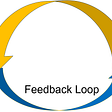## I Walk so Randomly on the Boulevard of Broken Links: Random Walks and Node Embeddings## 4 Tips You Need To Know on NLP — from a Twitter Data Scientist## CNN on GPU: MNIST Handwritten Digits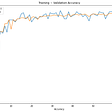## Enter the j(r)VAE: divide, (rotate), and order… the cards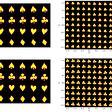## Learning from unlabelled data with COVID-19 Open Research Dataset## Object Detection with Fritz AI Studio## How to get a grip on Cross Validations## Mohammed Zeeshan Mulla

Data Science. Making Sense out of Data. Do the right n leave the rest.. All Views Are My Own ✌ | https://zeeshanmulla.me

## Electrical Power Systems — exploring smart cities via Data Science## Paradigms of various LSTM Networks## Yes, it’s worthy: Einstein summation notation applied to Machine Learning.## Machine Learning Multiplication — A LSTM Network